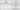Return On Investment – ROI Calculation & Its importance in Retail Operations

Retail ROI= Net Retail Margin ÷ Cost of Investment​

This is famously called an ROI working in sales fraternity. This is something that every retailer needs to understand before going ahead to start any project/store. This calculation happens with the cycle of 12 months into consideration but ideally, retailers should do with the complete calculation of five years before going ahead for any project. The following are the major bullet points that need to be taken care of while preparing your ROI sheet.

The five-year calculation is must

The entire calculation of ROI is supposed to happen with the tenure of five years keeping in mind as in initial 2-3 years we cover our Capex put in business and rest of the years it is the pure profit gets generate from business

Calculation of Depreciation

Depreciation is an accounting method of allocating the cost of a solid or physical asset over its useful life or life expectancy. Usually, depreciation gets calculated with tenure 60 months keeping in mind as we can see above that we have calculated ROI in vision with the five years and calculation of Depreciation is one of the main reason we calculate the ROI for next Five years from the date of inception & than each month cost for depreciation than can be taken in ROI as separate

Calculation of interest

It is being calculated in tenure of 12 Months. The interest can vary from 10%-14% for a case to case basis and depends on where you get your money to invest in a business but generally while calculating we calculate the same at the rate of 12%.

Each expense is Important

The common mistake which retailers do always is to ignore the small expenses like housekeeping, Credit Card, staff welfare, Festival donations, Etc.  ideally, these all should be taken into consideration while calculating ROI because as a whole in the end it makes a difference and capable to dent the margin by 3%-5%.

Understanding “Capex and Opex”

The above mumbo jumbo is meant “Capital Expenditure & Operational expenditure” respectively.Breakeven sales calculation

Again a very important calculation while preparing an ROI statement as we need to know what is the breakeven sales required from that store to recover the operational expenses including your depreciation and interest. This will also give clarity to measure your marketing exp required to push sales numbers.

EBITDA calculation

Earnings Before Interest, Taxes, Depreciation, and Amortization is a financial term used while preparing your final earning post all expenses as mentioned above. This is a measure used to calculate simple net income and is a clear cut profitability measurement.

EBITDA = Net Income + Interest +Taxes + D + A

Where:

D=Depreciation

A=Amortization​ (Amortization is paying off debt/Loan through interest and Principal in a set period)

Investment Limit

The right measurement of ROI can also bring you close to the perceptive of the “stock turnover ratio” of your business which will help you to assess your degree of investment in the business in terms of knowing where to stop investing the money…. The ideal ratio could be 1:4.

Finally, like many profitability measures, ROI simply highlights monetary gains when considering the returns on investment.

Thank You

Categories ROI

2 thoughts on “Return On Investment – ROI Calculation & Its importance in Retail Operations”

1.2.